# `` Earth and Sun '' website that can intuitively understand the rotation and revolution of the earth simply by operating with the mouse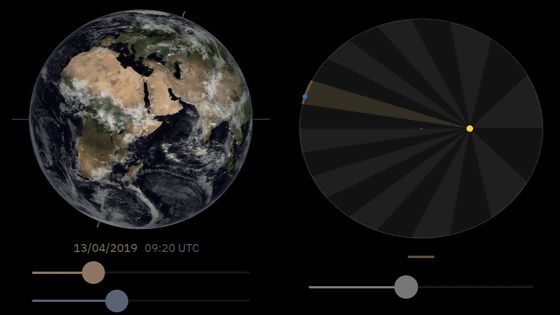A rotation that rotates around the earth earth's axis, on it is the content that is also learned in compulsory education junior high school doing revolution which rotates around the sun, the specific movement of the figure of the textbook is simplified It is difficult to understand intuitively. Engineer Bartosz Ciechanowski has released a demonstration that shows the rotation and revolution of the earth in one shot by simply operating the slider with the mouse.

Earth and Sun – Bartosz Ciechanowski

https://ciechanow.ski/earth-and-sun/

◆ Inclination of the earth axis
The earth's axis that connects the Earth's North and South Pole is the axis of rotation. The earth's axis is not perpendicular to the plane of revolution and is inclined about 23.4 degrees with respect to the axis of revolution. When turning, the part facing the sun is daytime, and the other side is night, but the demonstration on Earth and Sun clearly shows that the boundary between day and night is tilted with respect to the earth's axis. The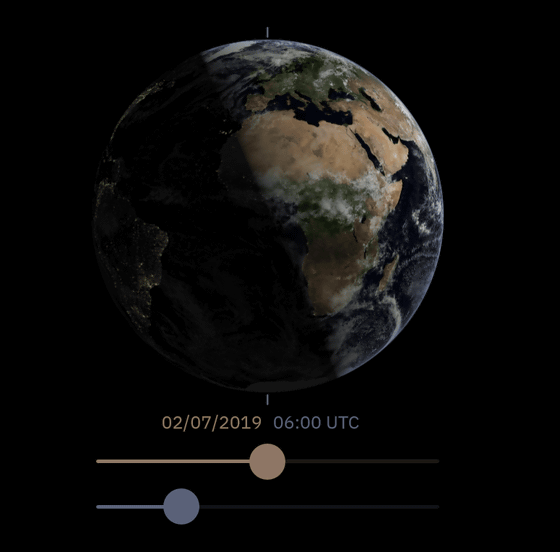Of the sliders below, the brown one can shift the date, the blue one can shift the time. If you actually shift it, you can understand how the place of light changes.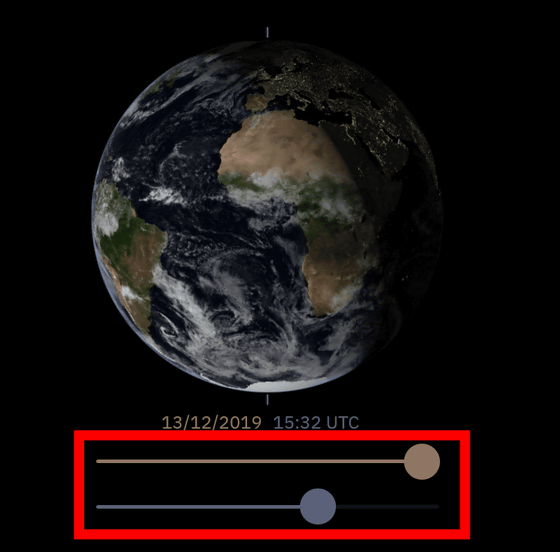In addition, the earth's axis itself is gradually moving under the influence of the gravitational force of the sun and the moon, and its direction changes with a period of approximately 25,772 years. This movement is called

Earth precession . Polaris of the Ursa Minor on the extension of the North Pole is recognized as a North Star at the time of writing the article, but as the earth's axis shifts, it gradually disappears from the North Star. In the following demo, you can check the trajectory of the earth axis by moving the slider.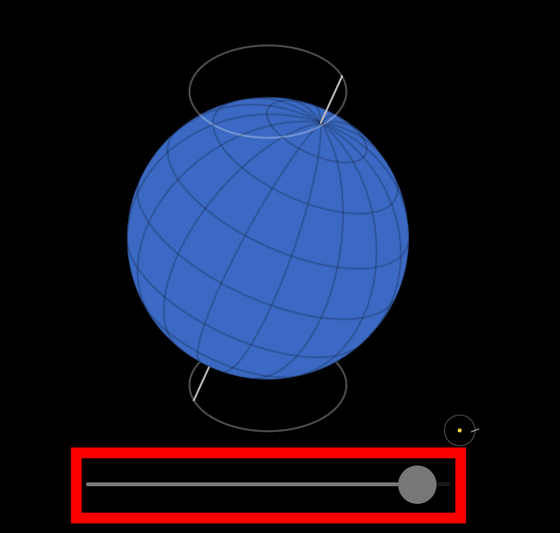Kepler's Law
In the textbook, there is a figure that “the earth is revolving around a sun and drawing a circular orbit”, but the actual orbit is not a “circle”. When you move the slider at the bottom of the figure, the earth (blue circle) turns around the sun (yellow circle), but you can clearly see that its orbit is not a circle but an ellipse.

This was discovered by Johannes Kepler , an astronomer of the 16th century, and summarized as ' Kepler's First Law '.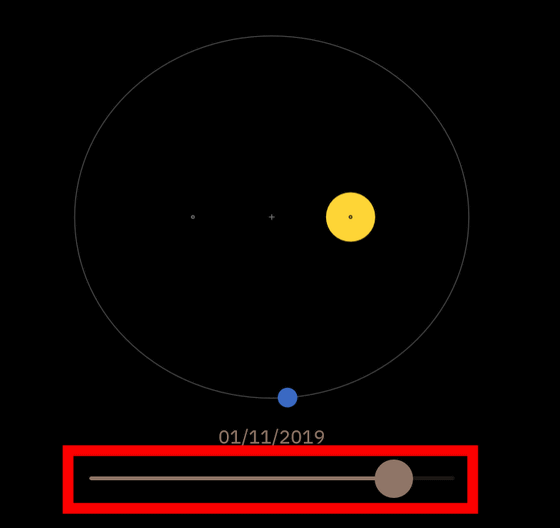Also, the speed at which the earth revolves around the sun is actually not constant. When the earth revolves around the sun, the following demo shows that the speed is not constant, `` Kepler's second law that the area drawn by the line connecting the planet and the sun per unit time is constant ' ' It is a visualization. By moving the slider, the earth (blue dot) moves in the orbit. In the demo figure, the orbital ellipse is divided like a pie according to the unit time through which the earth passes. It can be clearly seen that the distance that the earth revolves in the same unit time is shorter when the earth is away from the sun and longer when the earth is closer to the sun. In other words, depending on the distance between the earth and the sun, the revolution speed becomes slower or faster.

Star Day
It is generally considered that “the earth's rotation period is 24 hours”, but there is a strict deviation. For example, suppose the sun is just overhead at 12:00. At this time, in the following figure, the position of the arrow is where the observer is standing, and the direction of the arrow is the overhead direction.

Move the slider below to the right and the earth will revolve around the sun as it rotates. And the following is the state of the earth rotating 360 degrees, but the sun is not directly above when you see the position of the arrow.

When the slider was moved further to the right, the sun came right above the observer, and the next day was 12:00. In other words, the 24 hours used in our daily life is an apparent rotation period. The correct rotation period of the earth is called ' Star Day ', and it is actually about 23 hours 56 minutes 4 seconds. On the other hand, the apparent rotation period of 24 hours is said to be “ Sunday ”.

There are other demos on Ciechanowski's website that allow you to intuitively understand the movements of the earth and the sun.

in Science, Posted by log1i_yk